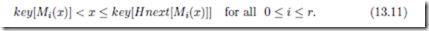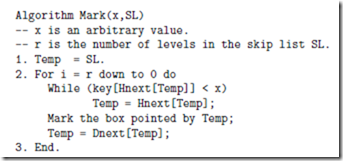# Randomized Dictionary Structures:Dictionary Operations.

###### Dictionary Operations

We shall use a simple procedure called Mark in all the dictionary operations. The procedure Mark takes an arbitrary value x as input and marks in each level the box containing the largest key that is less than x. This property implies that insertion, deletion or search should all be done next to the marked boxes. Let Mi(x) denote the box in the ith level that is marked by the procedure call Mark(x, SL). Recall the convention used in the linked structure that name of a box in a linked list is nothing but a pointer to that box. The keys in the marked boxes Mi(x) satisfy the following condition :The procedure Mark begins its computation at the box containing in level r. At any current level, we traverse along the level using horizontal pointers until we ﬁnd that the next box is containing a key larger or equal to x. When this happens, we mark the current box and use the descent pointer to reach the next lower level and work at this level in the same way.

The procedure stops after marking a box in level 0. See Figure 13.1 for the nodes marked by the call Mark(86,SL).We shall now outline how to use marked nodes for Dictionary operations. Let M0(x) be the box in level 0 marked by Mark(x). It is clear that the box next to M0(x) will have x iﬀ x S. Hence our algorithm for Search is rather straight forward.

To insert an item in the Skip List, we begin by marking the Skip List with respect to the value x to be inserted. We assume that x is not already in the Skip List. Once the marking is done, inserting x is very easy. Obviously x is to be inserted next to the marked boxes.

But in how many levels we insert x? We determine the number of levels by tossing a coin on behalf of x until we get a failure. If we get h successes, we would want x to be inserted in all levels from 0 to h. If h r, we simply insert x at all the existing levels and create a new level consisting of only and +that corresponds to the empty set. The insertion will be done starting from the base level. However, the marked boxes are identiﬁed starting from the highest level. This means, the Insert procedure needs the marked boxes in the reverse order of its generation. An obvious strategy is to push the marked boxes in a stack as and when they are marked in the Mark procedure. We may pop from the stack as many marked boxes as needed for insertion and then insert x next to each of the popped boxes. The deletion is even simpler. To delete a key x from S, simply mark the Skip List with respect to x. Note that if x is in Li, then it will be found next to the marked box in Li. Hence, we can use the horizontal pointers in the marked boxes to delete x from each level where x is present. If the deletion creates one or more empty levels, we ignore all of them and retain only one level corresponding to the empty set. In other words, the number of levels in the Skip List is reduced in this case. In fact, we may delete an item “on the ﬂy” during the execution of the Mark procedure itself. As the details are simple we omit pseudo codes for these operations.